### IMO Shortlist 1984 problem 8

Kvaliteta:
Avg: 0,0
Težina:
Avg: 0,0
Given points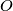$O$ and$A$ in the plane. Every point in the plane is colored with one of a finite number of colors. Given a point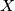$X$ in the plane, the circle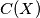$C(X)$ has center$O$ and radius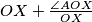$OX+{\angle AOX\over OX}$, where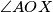$\angle AOX$ is measured in radians in the range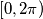$[0,2\pi)$. Prove that we can find a point$X$, not on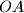$OA$, such that its color appears on the circumference of the circle$C(X)$.
Izvor: Međunarodna matematička olimpijada, shortlist 1984